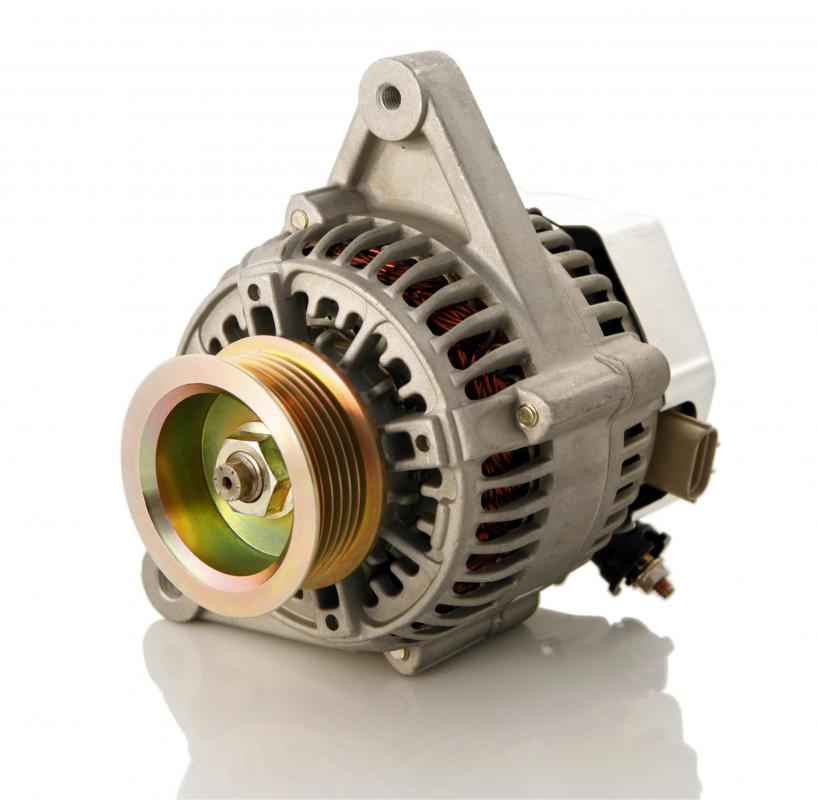# What is Mechanical Energy?

Daniel Liden

Mechanical energy is the sum of energy in a mechanical system, or any group of objects that interact based on basic mechanical principles. This includes both kinetic energy, the energy of motion, and potential energy, the stored energy of position. Typically, in a mechanical system, gravity is the only major outside force that needs to be considered. In a chemical system, by contrast, the forces between individual molecules and atoms must all be taken into account.An engine is an example of a system that produces mechanical energy.

#### General Background

Mechanical energy exists as both kinetic and potential energy in a system. Kinetic energy is present whenever an object is in motion. Potential energy is based on the position of an object; it is stored energy, and cannot do work on its own. It can, however, be converted to other forms of energy, including kinetic energy. A bowling ball suspended 10 feet (3 m) above the ground, for example, has no kinetic energy because it's not moving. It does, however, have a large amount of potential energy (in this case, gravitational potential energy) that would be converted to kinetic energy if the ball was allowed to fall.

High school physics classes often begin by instructing students about the basic principles of mechanical systems and their energy. This is because they are typically easier to visualize and easy to simplify. Basic calculations about these systems can be made without using calculus. In most simple physics problems, the mechanical system remains closed and factors that would normally remove energy from the system, such as friction and air resistance, are ignored.

#### How to Calculate Mechanical Energy

Total mechanical energy can be calculated simply by adding the potential and kinetic energy of the system. Potential energy (PE) is a product of the object's height above the ground (h), its mass (m), and the Earth's gravitational acceleration (g, which is 9.8 m/s2).

PE = h × m × g

An object's kinetic energy (KE) is the product of 1/2 its mass and its squared velocity (v).

KE = 1/2mv2

Mass is given in kilograms (kg), height in meters (m), velocity in meters per second (m/s), and energy is given in joules (j).

For example, the potential energy of a 5 kilogram (11 lbs) bowling ball that is 3 meters (10 ft) above the ground is 147 joules (5 kg × 3 m × 9.8 m/s2 = 147 j) whether the ball is in motion or at rest. If that ball is also falling at a speed of 2 m/s, its kinetic energy is 10 joules (1/2 × 5 kg × 22m/s = 10 j).

Once the potential and kinetic energy are known, then total mechanical energy can be found. The two types of energy are simply added together.

Mechanical Energy = PE + KE

In this example, the bowling ball's total mechanical energy is 157 joules (147 j + 10 j = 157 j).

#### Mechanical vs. Chemical and Nuclear Energy

There are many other forms of energy, and sometimes it can be difficult to properly distinguish one from the other. Chemical energy, for example, is that energy stored within chemical bonds in molecules. Nuclear energy is the energy present in interactions among particles in an atom's nucleus. Mechanical energy, in contrast, generally ignores the composition of objects and looks only at the objects in question, without concern for their molecular makeup.

This focus is designed to simplify calculations for mechanical energy and mechanical systems. Objects in these systems are usually treated as individual objects instead of as the sum of billions of molecules. Calculating both the kinetic and potential energy of a single object is a simple task; calculating these types of energy for billions of molecules would be extremely difficult. Without simplifying the parts in a mechanical system, scientists would need to examine the individual atoms and all of the interactions and forces existing between them. This is typically reserved for particle physics.

#### Conversion Between Types of Energy

Mechanical energy may be converted into other types of energy using special equipment. For example, generators are designed to take mechanical work and turn it into electricity. Other types of energy may also be converted into mechanical energy; for example, the internal combustion engine in a car converts the chemical energy in fuel to the mechanical energy used to make the car move.

## You might also Likeanon352808

What are mechanical energy characteristics?

anon351204

mechanical = potential + kinectic

anon334595

How would mechanical energy be related to tattoo machines?

anon319735

Yes, the gears on a bike are mechanical energy.

anon302029

Who first described or recognized mechanical energy?

anon221367

can you tell us the answer to the question, "how would i find the mechanical energy of an object in motion?"

anon151504

I'm trying to figure out how mechanical energy is related to electricity.

anon117333

What happens to the internal energy of the system when mechanical work is done on it?

anon83493

I am trying to figure out what classifies objects to make them be a part of the mechanical energy group. what certain characteristics makes certain objects classify as mechanical energy?

liden

Mechanical energy is the sum of kinetic and potential energy. Sure, a human is needed to put energy into the bicycle--but even the movement of human limbs is a form of mechanical energy. The way in which a bicycle moves would be a good example, then.

lokithebeak

I'm trying to come up with an easy example of mechanical energy for my 14yr old.

Are the gears on a bicycle considered mechanical energy? Or is that negated by the fact that a human is required to change it to kinetic energy?

Or maybe a wind farm instead, which would be using the kinetic energy of wind and NOT gravity though.

Can someone give me an easy example?